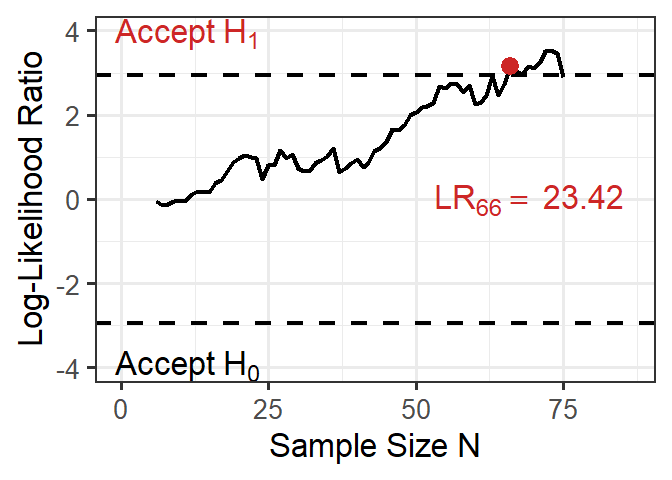## Overview

The sprtt package is a sequential probability ratio tests toolbox (sprtt).

The package contains:

• seq_ttest(), seq_anova() calculates sequential t-test and sequential one-way ANOVAs

• three data sets (df_income, df_stress, df_cancer) to run the examples in the t-test documentation

• plot_anova() plots results of sequential ANOVAs

• draw_sample_normal(), draw_sample_mixture() simulation of data sets

## Installation

### Release version from CRAN

This is the recommended version for a normal user.

# installs the package
install.packages("sprtt")

### Development version from GitHub

To get a bug fix or to use a feature from the development version, you can install the development version from GitHub.

# the installation requires the "devtools" package
# install.packages("devtools")
devtools::install_github("MeikeSteinhilber/sprtt")

## Documentation

Detailed documentation can be found on the home page. There are several articles covering the usage of the package, the theoretical background of the test, and also an extended use case.

Short examples can be found in the following paragraph.

### Quick Examples

Note

In the R code sections:

# comment: is a comment

function(): is R code

#> results of function(): is console output

# set seed --------------------------------------------------------------------
set.seed(333)

library(sprtt)

# t-TEST ----------------------------------------------------------------------
# one sample: numeric input ---------------------------------------------------
treatment_group <- rnorm(20, mean = 0, sd = 1)
results <- seq_ttest(treatment_group, mu = 1, d = 0.8)

# @ Operator
results@likelihood_ratio
#>  965.0728

# [] Operator
results["likelihood_ratio"]
#>  965.0728

# ANOVA -----------------------------------------------------------------------
# simulate data ---------------------------------------------------------------
set.seed(333)
data <- sprtt::draw_sample_normal(k_groups = 3,
f = 0.25,
sd = c(1, 1, 1),
max_n = 25)

# calculate sequential ANOVA --------------------------------------------------
results <- sprtt::seq_anova(y ~ x, f = 0.25, data = data, plot = TRUE)
# test decision
results@decision
#>  "continue sampling"
# test results
results
#>
#> *****  Sequential ANOVA *****
#>
#> formula: y ~ x
#> test statistic:
#>  log-likelihood ratio = 2.892, decision = continue sampling
#> SPRT thresholds:
#>  lower log(B) = -2.944, upper log(A) = 2.944
#> Log-Likelihood of the:
#>  alternative hypothesis = -2.715
#>  null hypothesis = -5.607
#> alternative hypothesis: true difference in means is not equal to 0.
#> specified effect size: Cohen's f = 0.25
#> empirical Cohen's f = 0.4045074, 95% CI[0.129478, 0.6171581]
#> Cohen's f adjusted = 0.355
#> degrees of freedom: df1 = 2, df2 = 72
#> SS effect = 10.74731, SS residual = 65.68208, SS total = 76.42939
#> *Note: to get access to the object of the results use the @ or [] instead of the \$ operator.

# plot results -----------------------------------------------------------------
sprtt::plot_anova(results)